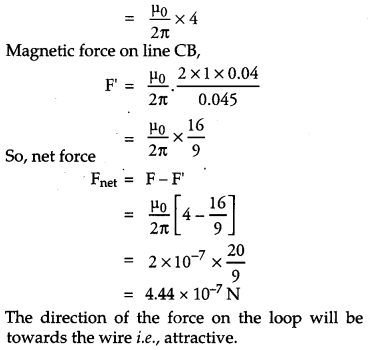## CBSE Previous Year Question Papers Class 12 Physics 2012 Delhi

### CBSE Previous Year Question Papers Class 12 Physics 2012 Delhi Set-I

Question 1.
When electrons drift in a metal from lower to higher potential, does it mean that all the free electrons of the metal are moving in the same direction ? 
No, when electric field is applied, the electrons will have net drift from lower to higher field but locally electrons may collide with ions and may change their direction of motion.

Question 2.
The horizontal component of the earth’s magnetic field at a place is B and angle of dip is 60°. What is the value of vertical component of earth’s magnetic field at equator ? 
On the equator, the value of both angle of dip (δ) and vertical component of earth’s magnetic field is zero. So, in this case,
Bv = 0.

Question 3.
Show on a graph, the variation of resistivity with temperature for a typical semiconductor. 
The following curve shows the variation of resistivity with temperature for a typical semiconductor.This is because, for a semiconductor, resistivity decreases rapidly with increasing temperature.

Question 4.
Why should electrostatic field be zero inside a conductor ? 
Charge on conductor resides on its surface. So if we consider a Gaussian surface inside the conductor to find the electrostatic field,
$$\phi=\frac{q}{\varepsilon_{0}}$$
Where, q = charge enclosed in Gaussian surface
q = 0, inside the conductor, Hence the electrostatic field inside the conductor is zero.

Question 5.
Name the physical quantity which remains same for microwaves of wavelength 1 mm and UV radiations of 1600 A in vacuum. 
Both microwaves and UV rays are a part of the electromagnetic spectrum. Thus, the physical quantity that remains same for both types of radiation will be their speeds which is equal to c (3 × 10 m/s).

Question 6.
Under what condition does a biconvex lens of glass having a certain refractive index act as a plane glass sheet when immersed in a liquid ? 
A biconvex lens will act like a plane sheet of glass if it is immersed in a liquid having the Same index of refraction as itself.

Question 7.
Predict the directions of induced currents in metal rings 1 and 2 lying in the same plane where current I in the wire is increasing steadily.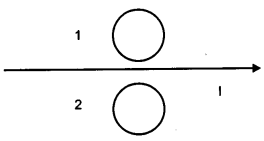Using Lenz’s law we can predict the direction of induced current in both the rings. Induced current oppose the cause of increase of magnetic flux. So,It will be clockwise in ring 1 and anticlockwise in ring 2.

Question 8.
State de-Broglie hypothesis. 
de Broglie postulated that the material particles may exhibit wave aspect. Accordingly a moving material particle behaves as wave and the wavelength associated with material particle is
$$\lambda=\frac{h}{m v}$$
where h = Planck’s constant
m = mass of the object
v = velocity of the object

Question 9.
A ray of light incident on an equilateral prism (μg = √3) moves parallel to the base line of the prism inside it. Find the angle of incidence for this ray. 
It is given that the prism is equilateral in shape. So, all the angles are equal to 60 . Thus, the angle of prism, A = 60
The angle of refraction in case of a prism,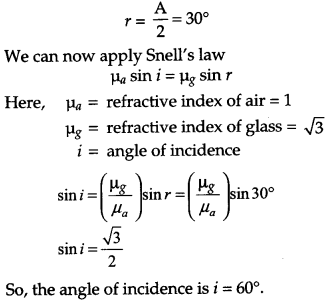Question 10.
Distinguish between ‘Analog and Digital signals. 
OR
Mention the functions of any two of the following used in communication system:
(i) Transducer
(ii) Repeater
(iii) Transmitter .
(iv) Band pass Filter

Question 11.
A cell of emf E and internal resistance r is connected to two external resistance Ri and R2 and a perfect ammeter. The current in the circuit is measured in four different situations
(i) without any external resistance in the circuit
(ii) with resistance R1 only
(iii) with R1 and R2 in series combination
(iv) with R1 and R2 in parallel combination
The currents measured in the four cases are 0.42 A, 1.05 A, 1.4 A and 4.2 A, but not necessarily in the order. Identify the currents corresponding to the four cases mentioned above.
The current relating to corresponding situations is as follows:
(i) Without any external resistance in the circuit:
$$\mathrm{I}_{1}=\frac{\mathrm{E}}{r}$$
The current in this case would be maximum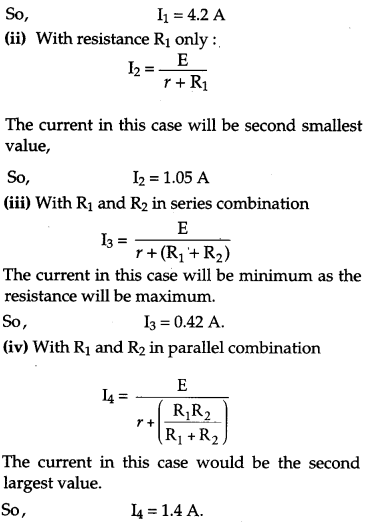Question 12.
The susceptibility of a magnetic material is -2.6 x 10-5. Identify the type of magnetic material and state its two properties. 
Answer : Diamagnetic materials have negative susceptibility. So the given magnetic material is diamagnetic.
Two properties of diamagnetic material:

1. They do not obey Curie’s law.
2. They are feebly repelled by a magnet.

Question 13.
Two identical circular wires P and Q each of radius R and carrying current I are kept in perpendicular planes such that they have a common center as shown in the figure. Find the magnitude and direction of the net magnetic field at the common centre of the two coils. 
Magnetic field produced by the two coils at their common centre having currents I1 and I2, radius a1 and a2, number of turns N1 and N2 respectively are given by :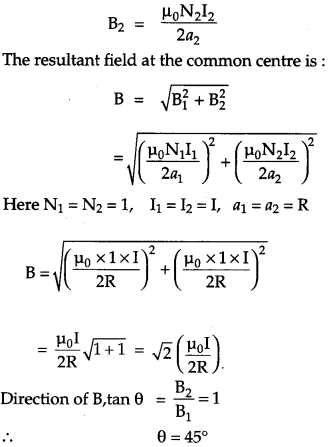Hence, the net magnetic field is directed at an angle of 45° with either of the fields.

Question 14.
When an ideal capacitor is charged by a d.c. battery, no current flows. However, when an a.c. source is used, the current flows continuously. How does one explain this based on the concept of displacement current ? 
When an ideal capacitor is charged by d.c. battery, charge flows till the capacitor gets fully charged.
When an a.c. source is connected then conduction current  $$i_{c}=\frac{d q}{d t}$$ flows in the connecting wire.
Due to changing current, charge deposited on the plates of the capacitor changes with time. Changing charge causes electric field between the plates of capacitor to be varying, giving rise to displacement current $$i_{c}=\frac{d q}{d t}$$
[As displacement current is proportional to the rate of flux variation].
Between the plates, electric field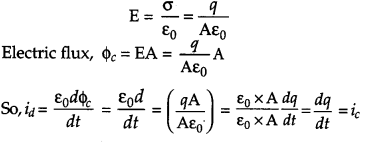Displacement current brings continuity in the flow of current between the plates of the capacitor

Question 15.
Draw a plot showing the variation of
(i) electric field (E) and
(ii) electric potential (V) with distance r due to a point charge Q. 
We know that for a point charge Q.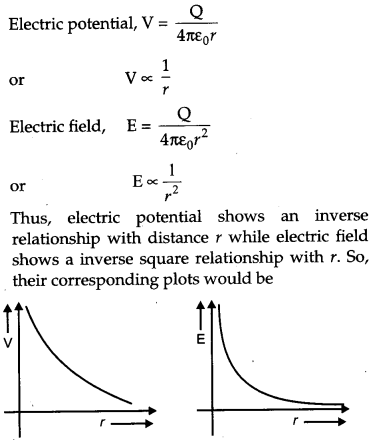Question 16.
Define self-inductance of a coil. Show that magnetic energy required to build up the current I in a coil of self inductance L is $$\frac{1}{2} \mathbf{L} \mathbf{I}^{2}$$ given
Self inductance is the inherent inductance of a circuit, given by the ratio of the electromotive force produced in the circuit by self-induction to the rate of change of current producing it. It is also called coefficient of self induction.
Suppose I = Current flowing in the coil at any time.
Φ= Amount of magnetic flux linked.
It is found that Φ ∝I
Φ = LI
where, L is the constant of proportionality and is called coefficient of self induction. SI unit of self-inductance is henry.
Let at t = 0, the current in the inductor is zero. So at any instant t, the current in the inductor is I and rate of growth of I is dl/dt.
Then, the induced emf is E = L x dl / dt
If the source is sending a constant current I
through the inductor for a small time dt, then small amount of work done by the source is given byThe total amount of work done by the source of emf, till the current increases from its initial value I = 0 to its final value I is given byThis work done by the source of emf used in building up current from zero to I is stored in the inductor in energy form. Therefore, energy stored in the inductor is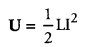Question 17.
The current in the forward bias is known to be more (~ mA) than the current in the reverse bias (~ µA). What is the reason, then, to operate the photo diode in reverse bias ? 
The current in the forward bias is due to majority carriers whereas current in the reverse bias is due to minority carriers. So current in forward bias is more (~mA) than current in reverse bias (~µA).

On illumination of photo diodes with light, the fractional change in the majority carriers would be much less than that in minority carriers. It implies that fractional change due to light on minority carriers dominated reverse bias current is more easily measurable than fractional change in forward bias current. So, photo diodes are operated in reverse bias condition.

Question 18.
A metallic rod of ‘L’ length is rotated with angular frequency of ‘ω’ with one end hinged at the center and the other end at the circumference of a circular metallic ring of radius L about an axis passing through the center and perpendicular to the plane of the ring. A constant and uniform magnetic field B parallel to the axis is present everywhere. Deduce the expression for the emf between the center and the metallic ring. 
The induced emf,Question 19.
The figure shows a series LCR circuit with L = 5.0 H, C = 80 µF, R = 40 Ω connected to a variable frequency 240 V source, calculate 
(i) the angular frequency of the source which drives the circuit at resonance,
(ii) the current at the resonating frequency,
(iii) the rms potential drop across the inductor at resonance.Answer: Given, L = 5.0 H, C = 80 μF, R = 40 Ω, V = 240 V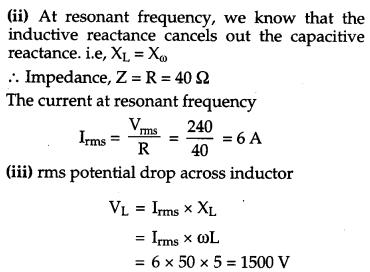Question 20.
A rectangular loop of wire of size 4 cm × 10 cm carries a steady current of 2 A. A straight long wire carrying 5 A current is kept near the loop as shown. If the loop and the wire are coplanar, find 
(i) the torque acting on the loop and
(ii) the magnitude and direction of the force on the loop due to the current carrying wire.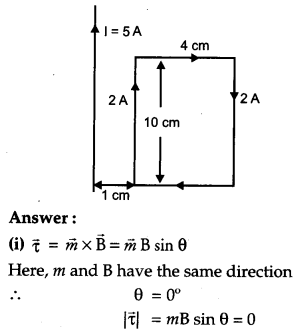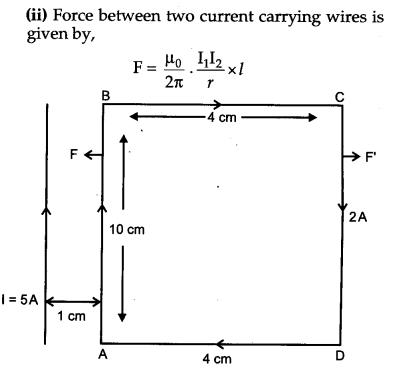Question 21.
(a) Using Bohr’s second postulate of quantization of orbital angular momentum show that the circumference of the electron in the nth orbital state in hydrogen atom is n times the de Broglie wavelength associated with it.
(b) The electron in hydrogen atom is initially in the third excited state. What is the maximum number of spectral lines which can be emitted when it finally moves to the ground state ? 
(a) According to Bohr’s second postulate of quantization, the electron can revolve around the nucleus only in those circular orbits in which the angular momentum of the electron is integral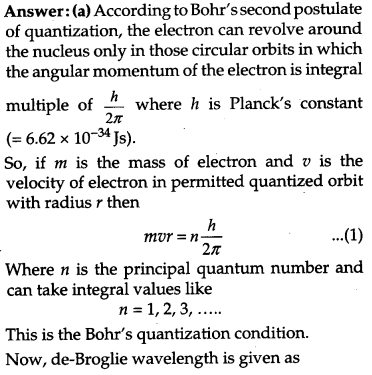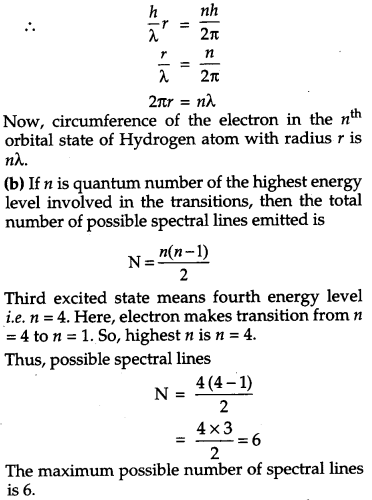Question 22.
In the figure a long uniform potentiometer wire AB is having a constant potential gradient along its length. The null points for the two primary cells of emfs ∈1 and ∈2 connected in the manner shown are obtained at a distance of 120 cm and 300 cm from the end A. Find
(i) ∈1/∈2 and
(ii) position of null point for the cell ∈1. 
How is the sensitivity of a potentiometer increased ?
OR
Using Kirchhoff’s rules determine the value of unknown resistance R in the circuit so that no current flows through 4 Ω resistance. Also find the potential between A and D.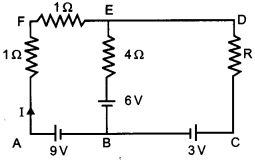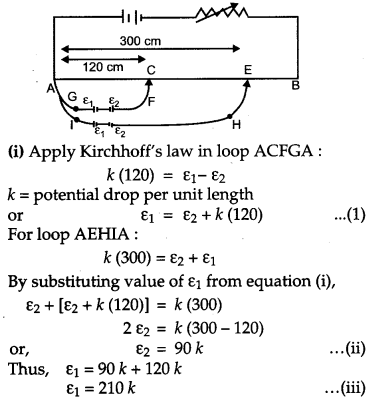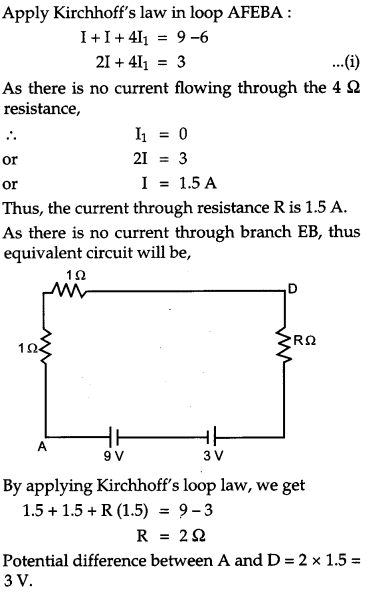Question 23.
(i) What characteristic property of nuclear force explains the constancy of binding energy per nucleon (BE/A) in the range of mass number ‘A’ lying 30 < A < 170 ?
(ii)Show that the density of nucleus over a wide range of nuclei is constant and independent of mass number A. 
(i) The constancy of BE/A over most of the range is due to saturation property of nuclear force. In heavy nuclei: nuclear size > range of nuclear force. So, nucleons experiences nearly constant interaction.
(ii) To find the density of nucleus of an atom, we have an atom with mass number let say A and let mass of the nucleus of the atom of the mass number A be m A.
Where, m is mass of one nucleon.
Let radius of nucleus be R.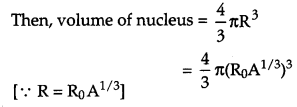This expression is independent of mass number A and since m, π and R0 have same values for any atom, therefore, density is constant also.

Question 24.
Write any two factors which justify the need for modulating a signal. Draw a diagram showing an amplitude modulated wave by superposing a modulating signal over a sinusoidal carrier wave. 

Question 25.
Write Einstein’s photoelectric equation. State clearly how this equation is obtained using the photon picture of electromagnetic radiation. Write the three salient features observed in photoelectric effect which can be explained using this equation.
Einstein’s photoelectric equation,
hv = Kmax + Φ
Where, h = Planck’s constant
Φ = Work function

According to Planck’s quantum theory, light radiations consist of small packets of energy. Einstein postulated that a photon of energy hv is absorbed by the electron of the metal surface, then the energy equal to Φ is used to liberate electron from the surface and rest of the energy hv – Φ becomes the kinetic energy of the electron.
Energy of photon is,
E = hv
The minimum energy required by the electron of a material to escape out of its work function ‘Φ’. The additional energy acquired by the electron appears as the maximum kinetic energy ‘Kmax‘ of the electron.

i.e, Kmax = hv – Φ
or hv = Kmax + Φ
where Φ = eV0

Sailent features observed in photoelectric effect:

1. The stopping potential and hence the maximum kinetic energy of emitted electrons varies linearly with the frequency of incident radiation.
2. There exists a minimum cut-off frequency V0, for which the stopping potential is zero.
3. Photoelectric emission is instantaneous

Question 26.
(a) Why are coherent sources necessary to produce a sustained interference pattern ?
(b)In Young’s double slit experiment using monochromatic light of wavelength λ, the intensity of light at a point on the screen where path difference is λ, is K units. Find out the intensity of light at a point where path difference is λ/3. 
(a) Coherent sources have constant phase difference between them i.e., phase difference does not change with time. Hence, the intensity distribution on the screen remains constant and sustained.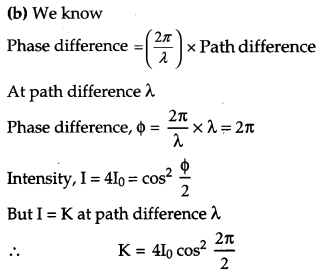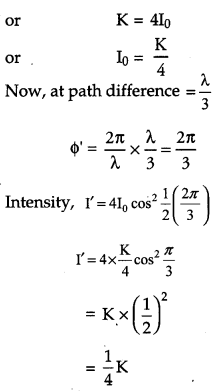Question 27.
Use Huygens’s principle to explain the formation of diffraction pattern due to a single slit illuminated by a monochromatic source of light. When the width of the slit is made double the original width, how would this affect the size and intensity of the central diffraction band ? 
Consider a parallel beam of monochromatic light is incident normally on a slit of width b as shown in figure. According to Huygens’s principle, every point of slit acts as a ‘ source of secondary wavelets spreading in all directions. Screen is placed at a larger distance.

Consider a particular point P on the screen that ; receives waves from all the secondary sources. All these waves start from different point of the slit and interfere at point P to give resultant intensity.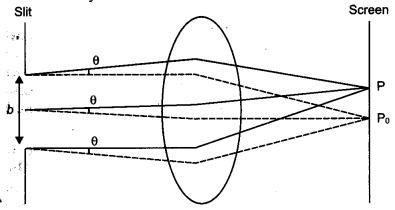Point Po is a bisector plane of the slit. At Po, all waves are travelling equal optical path. So all wavelets are in phase thus interfere constructively with each other and maximum intensity is observed. As we move from Po, the wave arrives with different phases and intensity is changed. Intensity at point P is given by
$$\mathrm{I}=\mathrm{I}_{0} \frac{\sin ^{2} \alpha}{\alpha}$$

$$\text { Where } \alpha=\frac{\pi}{\lambda} b \sin \theta$$
For central maxima, α= 0 thus,
I = Io
When the width of slit is made double the original width intensity will get four times of its original value.
Width of central maximum is given by,
$$\beta=\frac{2 \mathrm{D} \lambda}{b}$$
Where, D = Distance between screen and slit,
λ = Wavelength of the light,
b = size of slit
So with the increase in size of slit the width of central maxima decrease. Hence, double the size of the slit would result in half the width of the central maxima.

Question 28.
Explain the principle of a device that can build up high voltage of the order of a few million volts. Draw a schematic diagram and explain the working of this device. Is there any restriction on the upper limit of the high voltage set up in this machine ? Explain. 
OR
(a) Define electric flux. Write its S.I. units.
(b) Using Gauss’s law, prove that the electric field at a point due to a uniformly charged infinite plane sheet is independent of the distance from it.
(c) How is the field directed if
(i) the sheet is positively charged,
(ii) negatively charged ?
Van de Graaff generator is the device used for building up high voltages of the order of a few million volts. Such high voltages are used to accelerate charged particles such as electrons, protons, ions, etc.

It is based on the principle that charge given to a hollow conductor is transferred to outer surface and is distributed uniformly over it.
Construction:It consists of large spherical conducting shell (S) supported over the insulating pillars. A long narrow belt of insulating material is wound – around two pulleys P1 and P2, B1 and B2 are two sharply pointed metal combs. B1 is called the spray comb and B2 is called the collecting comb. Working : The spray comb is given a positive potential by high tension source. The positive charge gets sprayed on the belt.

As the belt moves and reaches the sphere, a negative charge is induced on the sharp ends of collecting comb-B2 and an equal positive charge is induced on the farther end of B2.

This positive charge shifts immediately to the outer surface of S. Due to discharging action of sharp points of B2, the positive charge on the belt is neutralized. The uncharged belt returns down and collects the positive charge from B1, which in turn is collected by B2. This is repeated. Thus, the positive charge of S goes on accumulating. In this way, potential differences of as much as 6 or 8 million volts (with respect to the ground) can be built up. The main limiting factor on the value of high potential is the radii of sphere.

If the electric field just outside the sphere is sufficient for dielectric breakdown of air, no more charge can be transferred to it.
For a conducting sphere,
Electric field just outside sphere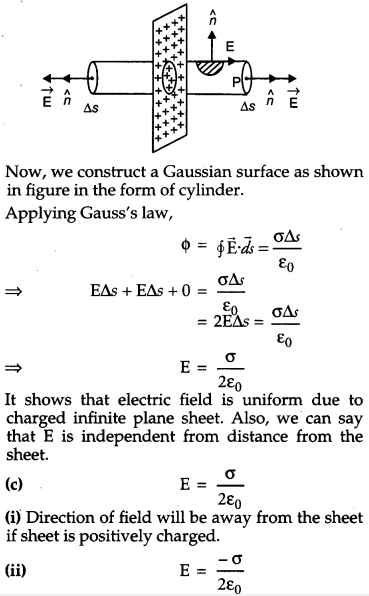Direction of field will be towards the sheet if sheet is negatively charged.

Question 29.
Define magnifying power of a telescope. Write its expression. A small telescope has an objective lens of focal length 150 cm and an eye-piece of focal length 5 cm. If this telescope is used to view a 100 m high tower 3 km away, find the height of the final image when it is formed 25 cm away front the eye-piece. 
OR
How is the working of a telescope different from that of a microscope ? The focal lengths of the objective and eyepiece of a microscope are 1.25 cm and 5 cm respectively. Find the position of the object relative to the objective in order to obtain an angular magnification of 30 in normal adjustment.
Magnifying power of a telescope is defined as the ratio of the angle subtended at the eye by the image formed at the least distance of distinct vision to the angle subtended at the eye by the object lying at infinity, when seen directly.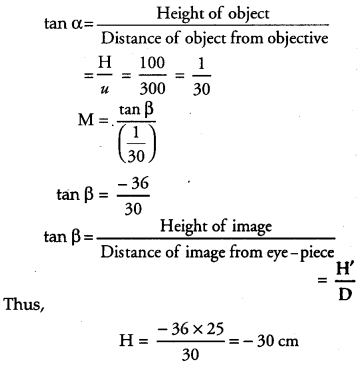OR
A microscope is used to look into smaller objects like structure of cells etc. On the other hand, a telescope is used to see larger objects that are very far away like stars, planets etc.

Telescope mainly focuses on collecting the light into the objective lens, which should thus be large, where the microscope already has a focus and the rest is blurred around it. There is a big difference in their magnification factors. For telescope, the angular magnification is given by
$$\mathrm{M}=\frac{f_{o}}{f_{e}}$$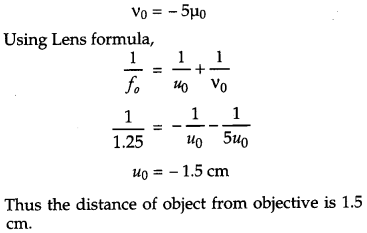Question 30.
Draw a simple circuit of a CE transistor amplifier. Explain its working. Show that the voltage gain, Av, of the amplifier is given by  $$\mathbf{A}_{\mathbf{V}}=\frac{-\beta_{a c} \mathbf{R} \mathbf{L}}{r_{i}}$$
where βac is the current gain,
‘ RL’ is the load resistance and,
‘ ri ‘ is the input resistance of the transistor.
What is the significance of the negative sign in the expression for the voltage gain ? 
OR
(a) Draw the circuit diagram of a full wave rectifier using p-n junction diode. Explain its working and show the output, input wave forms.
(b) Show the output wave forms (Y) for the following inputs A and B of
(i) OR gate
(ii) NAND gate### CBSE Previous Year Question Papers Class 12 Physics 2012 Delhi Set-II

Note : Except for the following questions, all the remaining questions have been asked in previous Set.

Question 1.
Why must electrostatic field be normal to the surface at every point of a charged conductor ? 
The electrostatic field must be normal to the surface of the conductor at every point because if the electric field is not normal to the surface of the charged conductor, there will be a component of the electric field along the surface of the conductor, which would exert a force on the charges at the surface. Due to this, charge starts flowing which is not possible.

Question 2.
Predict the direction of induced current in a metal ring when the ring is moved towards a straight conductor with constant speed v. The conductor is carrying current I in the direction shown in the figure.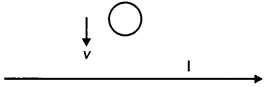Clockwise

Question 3.
Derive the expression for the self inductance of a long solenoid of cross-sectional area A and length l, having n turns per unit length. 
Self-inductance of a long Solenoid :
The magnetic field B at any point inside a solenoid is constant and given by,Question 4.
Two identical circular loops, P and Q, each of radius r and carrying currents I and 21 respectively are lying in parallel planes such that they have a common axis. The direction of current in both the loops is clockwise as seen from O which is equidistance from the both loops. Find the magnitude of the net magnetic field at point O.Question 5.
A series LCR circuit with L = 4.0 H, C = 100 μF, R = 60 Ω connected to a variable frequency 240 V source as shown in figure calculate : 
(i) the angular frequency of the source which drives the circuit at resonance,
(ii) the current at the resonating frequency,
(iii) the rms potential drop across the inductor at resonance.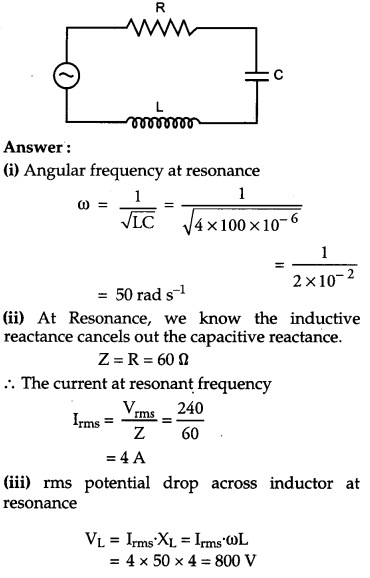Question 6.
A rectangular loop of wire of size 2 cm × 5 cm carries a steady current of 1 A. A straight long wire carrying 4 A current is kept near the loop as shown in the figure. If the loop and the wire are coplanar, find
(i) the torque acting on the loop and
(ii) the magnitude and direction of the force on the loop due to the current carrying wire.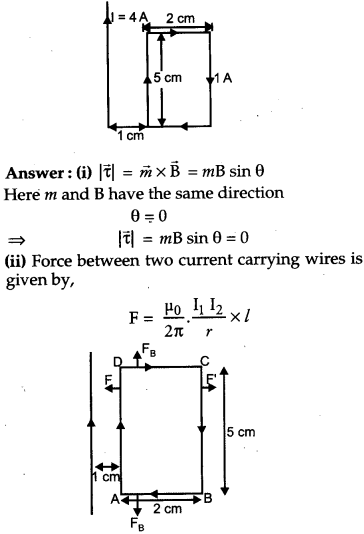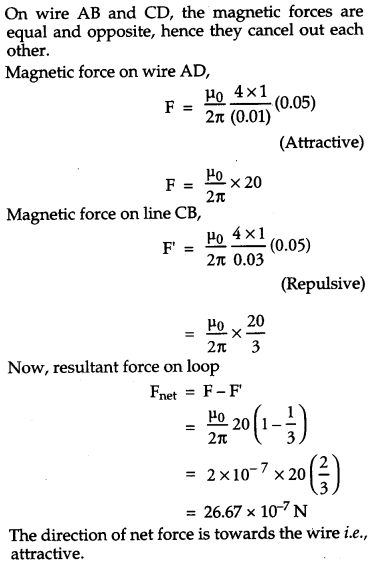Question 7.
Name the three different modes of propagation of electromagnetic waves. Explain, using a proper diagram the mode of propagation used in the frequency range above 40 MHz. 

### CBSE Previous Year Question Papers Class 12 Physics 2012 Delhi Set-III

Note : Except for the following questions, all the remaining questions have been asked in previous Set

Question 1.
Why is electrostatic potential constant throughout the volume of the conductor and has the same value (as inside) on its surface ? 
We know that the electric field inside a conductor is zero. This is the reason why electrostatic potential is constant, as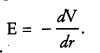Question 2.
Predict the direction of induced current in metal rings 1 and 2 when current I in the wire is steadily decreasing ?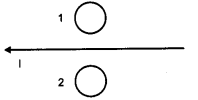Using Lenz’s law, we can predict the direction of induced current in the ring. Induced current oppose the cause of change of magnetic flux in moving towards the conductor.

In metal Ring 1, induced current will be in is clockwise.

In metal Ring 2, induced current will be in is anticlockwise.

Question 3.
The relative magnetic permeability of a magnetic material is 800. Identify the nature of magnetic material and state its two properties. 
A magnetic material having relative permeability of 800 would be classified as a ferromagnet. A few examples of such materials include iron and nickel.
Its two properties are :

1. All ferromagnetic materials become para-magnetic when heated to a temperature above the Curie temperature (Tc).
2. These materials show a strong attraction towards magnetic fields and have a tendency to become magnets themselves.

Question 4.
Two identical circular loops, P and Q, each of radius r and carrying equal currents are kept in the parallel planes having a common axis passing through O. The direction of current in P is clockwise and in Q is anti-clockwise as seen from O which is equidistant from the loops P and Q. Find the magnitude of the net magnetic field at O.Now, as the current flowing in loop P is clockwise, by using right hand thumb’s rule, the direction of the magnetic field will be towards left and as the current in loop Q is clockwise then the direction of magnetic field is towards left. So the net magnetic field at point O will be the sum of the magnetic fields due to loops P and Q.
So, net fieldQuestion 5.
A rectangular loop of wire of size 2.5 cm × 4 cm carries a steady current of 1 A. A straight wire carrying 2 A current is kept near the loop as shown. If the loop and the wire are co-planar, find the
(i) torque acting on the loop and
(ii) the magnitude and direction of the force on the loop due to the current carrying wire.